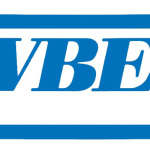# Excel Workbook.BeforeClose EventExcel Workbook_BeforeClose Event Excel predefines some popular actions that  you would do on different Objects (worksheet, workbook, button, etc), those actions are called Event. For example, activating a worksheet is an Event, closing a workbook is an Event, clicking on a button is an event. Each Object has its own list of Events, Workbook has a list of Events (e.g. close workbook, open workbook), worksheet has a list of Events (e.g. activate worksheet, edit a Cell). If you perform an Event...

# Excel Workbook.Deactivate EventExcel Workbook_Deactivate Event Excel predefines some popular actions that  you would do on different Objects (worksheet, workbook, button, etc), those actions are called Event. For example, activating a worksheet is an Event, closing a workbook is an Event, clicking on a button is an event. Each Object has its own list of Events, Workbook has a list of Events (e.g. close workbook, open workbook), worksheet has a list of Events (e.g. activate worksheet, edit a Cell). If you perform an Event,...

# Excel Workbook.Activate EventExcel Workbook_Activate Event Excel predefines some popular actions that  you would do on different Objects (worksheet, workbook, button, etc), those actions are called Event. For example, activating a worksheet is an Event, closing a workbook is an Event, clicking on a button is an event. Each Object has its own list of Events, Workbook has a list of Events (e.g. close workbook, open workbook), worksheet has a list of Events (e.g. activate worksheet, edit a Cell). If you perform an Event, s...

# Function, Sub, Method, Property DifferencesThis tutorial explains differences among Function, Sub, Method, Property Excel / Access VBA. You may also want to read: Difference between Dim and Set Excel VBA Option Explicit to force declaration of variables Difference between ByRef ByVal Function, Sub, Method, Property Differences In my blog, I have categorized different VBA topics by their properties. If you don't understand Function, Sub, Method, Property Differences, you will find my tips confusing and difficult to use. Thos...

# Excel Find FunctionThis Excel tutorial explains how to use Excel FIND Function for Excel worksheet, and also explains the differences between INSTR and SEARCH function. You may also want to read: Extract text in brackets Remove text in brackets Excel Find Function Excel Find function is used to search a substring (part of the string) within a string and return the position of the first occurrence, note that Excel Find function is case sensitive. Excel Search function is very similar to Find functi...

# Excel Search FunctionThis Excel tutorial explains the Excel Search Function for Excel worksheet, and also explains the differences between INSTR and FIND function. Excel Search Function Excel Search function allows Wildcard to search a substring (part of the string) within a string and return the position of the first occurrence. Excel Search Function is not case sensitive, you should consider using Excel FIND Function for case sensitive comparison, but FIND Function cannot use Wildcard. For example, ...

# Change Pivot Table Layout using VBAChange Pivot Table Layout, why? To illustrate why we want to change Pivot Table Layout, lets see the below example. Assume that we use the following data to create a Pivot Table. We want to show the total summary, group by Department, Empl ID. Excel 2003 - Tabular layout Pivot Table layout is called "Tabular", where each grouping is in one column, and the subtotal is at the bottom of each group. Excel 2007 and onwards - Compact layout By default, Pivot Table layout is called ...

# Create Pivot Table using Excel VBAThis Excel tutorial explains how to create Pivot Table using Excel VBA, set Print Area for Pivot Table Create Pivot Table using Excel VBA Create Pivot Table using Excel VBA is very straight forward and easy to use. If you don't understand any Object or Property that VBA Pivot Table uses, simply use "Record Macro" function to do recording to see the underlying VBA code behind each action, such as create Pivot Table, change Pivot Table Fields, select Critera, delete Pivot Table, etc. In this ...